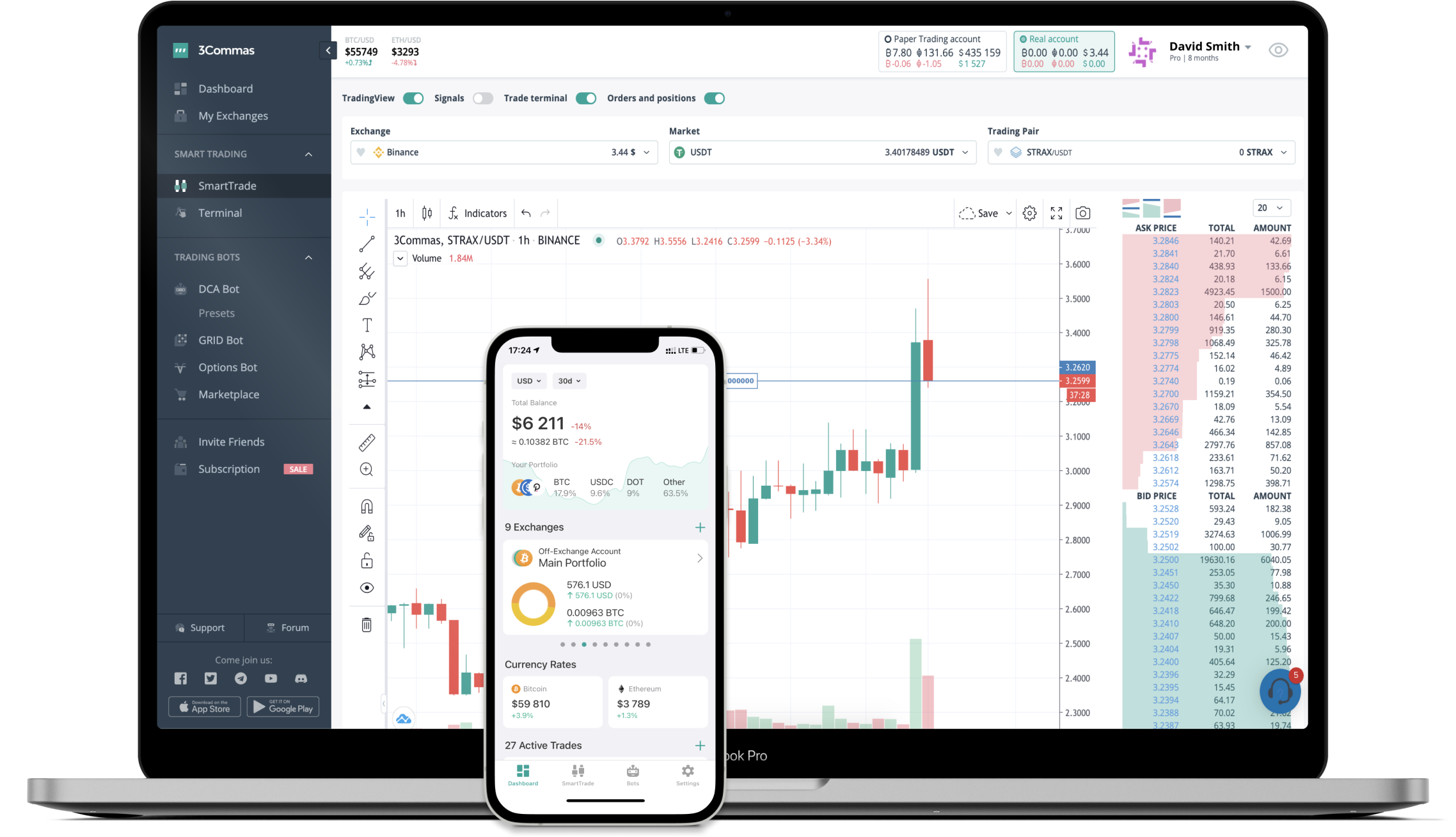# MODX to INR Converter

MODEL-X-coin
PriceINR 1.017
VolumeINR 79
How it work

The 3Commas currency calculator allows you to convert a currency from MODEL-X-coin (MODX) to Indian Rupee (INR) in just a few clicks at live exchange rates.

Simply enter the amount of MODEL-X-coin you wish to convert to INR and the conversion amount automatically populates. You can also use our Prices Calculator Table to calculate how much your currency is worth in other denominations, i.e. .1 MODX, .5 MODX, 1 MODX, 5 MODX, or even 10 MODX.

## MODX to INR Exchange Calculator

The chart displays MODEL-X-coin price changes in INR.

The ultimate tools for crypto traders to maximize trading profits while minimizing risk and loss.## Latest MODX to INR price calculator

MODEL-X-coin market price is updated every three minutes and is automatically displayed in INR. Below are the most popular denominations to convert to INR.

MODEL-X-coin
Indian Rupee
0.01
INR
0.10
INR
1.02
INR
2.03
INR
3.05
INR
5.09
INR
10.17
INR
25.43
INR
50.85
INR
101.70
INR
254.25
INR
508.50
INR
1017.00
INR
2542.50
INR
Indian Rupee
MODEL-X-coin
0.00983284
MODX
0.09832842
MODX
0.98328417
MODX
1.96656834
MODX
2.94985251
MODX
4.91642085
MODX
9.83284169
MODX
24.58210423
MODX
49.16420846
MODX
98.32841691
MODX
245.82104228
MODX
491.64208456
MODX
983.28416912
MODX
2458.21042281
MODX

## FAQ

### How much is 1 MODEL-X-coin in Indian Rupee?

MODEL-X-coin price in INR is constantly changing.

At this moment, 1 MODEL-X-coin equals 1.017 INR

### How do I calculate the conversion from MODX to INR?

The 3Commas MODEL-X-coin Calculator allows you to easily calculate the conversion price of MODX to INR by simply entering the amount of MODEL-X-coin in the corresponding field and will automatically convert the value in Indian Rupee (INR).

You can also use our MODEL-X-coin price table above to check the latest MODEL-X-coin price in major fiat and crypto currencies.

### How can I convert MODEL-X-coin to INR?

The most common way of converting MODX to INR is by using a Crypto Exchange or a P2P (person-to-person) exchange platform like LocalBitcoins, etc.